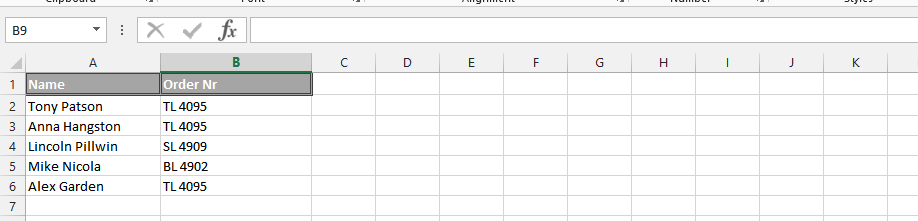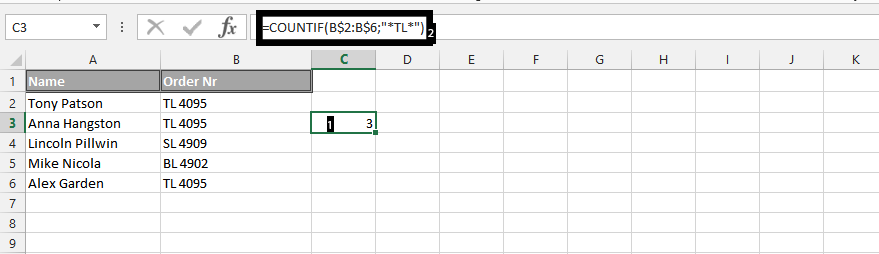#### How to Countif with Partial Match

In this Excel tutorial you will learn how to countif cells with partial match in Excel. The efficiency of using the countif partial text is its contribution to a productive end-result.

## Data preparation

This is a function that starts with having a data like this:## Countif formula

To count cells that contain a partial match in Microsoft Excel, you can use the COUNTIF function with the wildcard character “*”. Here’s how:

Click on an empty cell (1), and then type in the =COUNTIF(B\$2:B\$6,”*TL*”) to find the numbers of TL.The result will be the number of cells in the specified range that contain the partial match.

Note: This allows the ability to count the numbers of TL that are in the column b, and this is traditionally determined by the user, so it is up to the user to decide what he or she wants to look for.

This is how countif with approximate match is being done in Excel.

## Using the COUNTIF Function to Count Cells with a Partial Match in Other Columns

The COUNTIF function can be used to count cells with a partial match in any column. For example, the following formula will count all of the cells in the range A2:A6 that contain the text string “TL” anywhere in the cell:

``=COUNTIF(A\$2:A\$6,"*TL*")``

You can also use the COUNTIF function to count cells with a partial match in a range of columns. For example, the following formula will count all of the cells in the range A2:C6 that contain the text string “TL” anywhere in the cell:

``=COUNTIF(A\$2:C\$6,"*TL*")``

To use the COUNTIF function to count cells with a partial match, you need to specify the range of cells that you want to search and the text string that you are looking for. The text string can be any text string, including spaces and punctuation marks.

The wildcard character “*” can be used to represent any number of characters. For example, the formula `=COUNTIF(A\$2:A\$6,"TL")` will count all of the cells in the range A2:A6 that contain the text string “TL” anywhere in the cell.

The wildcard character “?” can be used to represent a single character. For example, the formula `=COUNTIF(A\$2:A\$6,"T?L")` will count all of the cells in the range A2:A6 that contain the text string “TL” where the second character is any single character.

## Other Ways to Count Cells with Partial Matches

In addition to the COUNTIF function, there are other ways to count cells with partial matches in Excel. Here are a few examples:

1. The FIND function can be used to find the position of a text string within a cell. For example, the formula `=FIND("TL", B2)` will return the position of the text string “TL” in cell B2. You can then use the COUNTIF function to count the number of cells that contain the text string “TL” at the specified position.
2. The LEFT function can be used to extract the leftmost characters from a text string. For example, the formula `=LEFT(B2, 2)` will extract the first two characters from cell B2. You can then use the COUNTIF function to count the number of cells that contain the text string “TL” as the first two characters.
3. The RIGHT function can be used to extract the rightmost characters from a text string. For example, the formula `=RIGHT(B2, 2)` will extract the last two characters from cell B2. You can then use the COUNTIF function to count the number of cells that contain the text string “TL” as the last two characters.